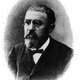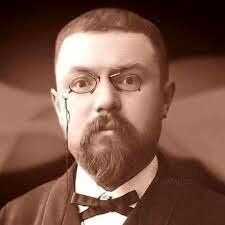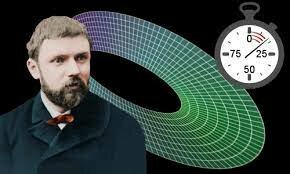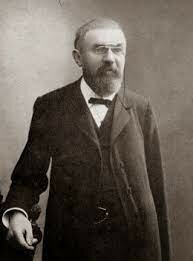• # Henri Poincare - New Branch of MathematicsBetween the years of 1881-1882 Henri Poincare had created a new branch of mathematics, called qualitative theory of differential equations. This studies the behavior of differential equations by ways other than finding their solutions. Poincare showed others how it is possible gather the most important information about the behavior of a family of solutions without having to completely solve the equation itself. This was used to approach problems in mathematical physics as well as in mechanics.
• # Henri Poincare "Three-Body Problem"At the age of 32, Henri Poincare had been elected to the French Academy of Sciences, he then won Oscar II, King of Sweden's mathematical competition for a resolution of the "three-body problem" concerning the free motion of multiple orbiting bodies. Three-body problem is finding the general solution to the motion of two or more orbiting bodies that are in a solar system. This problem had stumped mathematicians since Newton's time, until Poincare had solved it. https://youtu.be/et7XvBenEo8
• # Henri Poincare - TopologyHenri Poincare is considered to be one of the founders of the field of topology. Poincare's research in geometry eventually let him to the topological definition of homotopy and homology. This provided a formula which related vertices and faces, and the number of edges of n-dimensional polyhedron which resulted in the first ever precise formulation of the intuitive notion of dimension.
• # Henri Poincare - relation between mass and energyIn 1900, Poincare had discovered a relation between mass and electromagnetic energy. He can learned that the electromagnetic field has its own momentum, and that the action/reaction principle does not hold for only matter. Poincare had realized this when he tried to determine if the center of gravity would still move with a uniform velocity if electromagnetic fields were included.